# Knowledge Base

## Solution 11815: Finding a Maximum or Minimum of a Function on a TI-89 Family, TI-92 Family, and Voyage™ 200 Graphing Calculator.

### How can I find the maximum or minimum value of a function using a TI-89 family, TI-92 family, or Voyage 200?

To calculate a maximum or minimum of a function, access the Math menu after graphing a function. Then, from the menu that appears, select the calculation to be performed.

The example below will demonstrate how to find a maximum of a function.

Please Note: The function graphed must actually have a maximum or minimum in order for it to be calculated.

To find the maximum of the function -X2+X+6:

1) Press [◊] [Y=].
2) In an open entry line, press [(-)] [X] [^]  [+] [X] [+] .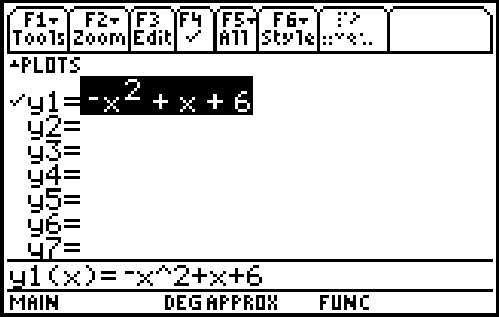3) Press [◊] [GRAPH].
4) Press [F5] .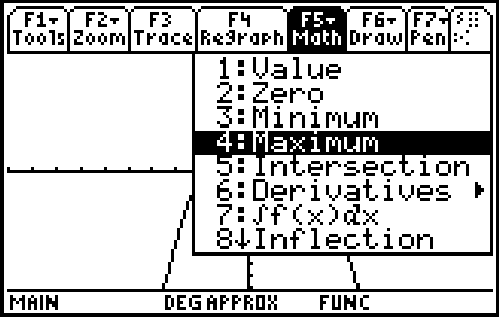5) Specify the lower bound for xc by either moving the trace cursor to the left of the vertex (maximum point), or by directly inputting a value.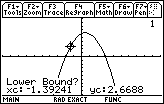6) Press [ENTER].
7) Next, a prompt for "Upper Bound?" is displayed.
8) Specify the upper bound for xc by either moving the trace cursor to the left of the vertex, or by directly inputting a value.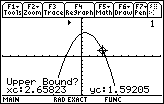9) Press [ENTER].The value of the maximum (the vertex) is then displayed on the screen.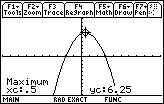The steps above are the same used when calculating minimums, zeros, inflections, and intersections.

Please see the TI-89 family, TI-92 family and Voyage 200 guidebooks for additional information.